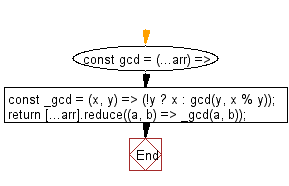# JavaScript: Calculate the greatest common divisor between two or more numbers/arrays

## JavaScript fundamental (ES6 Syntax): Exercise-223 with Solution

Write a JavaScript program to calculate the greatest common divisor between two or more numbers/arrays.

• The inner _gcd function uses recursion.
• Base case is when y equals 0. In this case, return x.
• Otherwise, return the GCD of y and the remainder of the division x/y.

Sample Solution:

JavaScript Code:

``````//#Source https://bit.ly/2neWfJ2
const gcd = (...arr) => {
const _gcd = (x, y) => (!y ? x : gcd(y, x % y));
return [...arr].reduce((a, b) => _gcd(a, b));
};
console.log(gcd(8, 36));
console.log(gcd(...[12, 8, 32]));
```
```

Sample Output:

```4
4
```

Pictorial Presentation:Flowchart:Live Demo:

See the Pen javascript-basic-exercise-223-1 by w3resource (@w3resource) on CodePen.

Improve this sample solution and post your code through Disqus

What is the difficulty level of this exercise?

Test your Programming skills with w3resource's quiz.

﻿